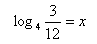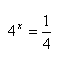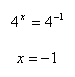Method 1
Example 2

Solve the equation log 43 - log 412 = x
Step 1. Use properties to convert to a single log.Step 2. Translate the log equation to its equivalent exponential form.Step 3. If needed, change the two expressions to the same base and solve for x.